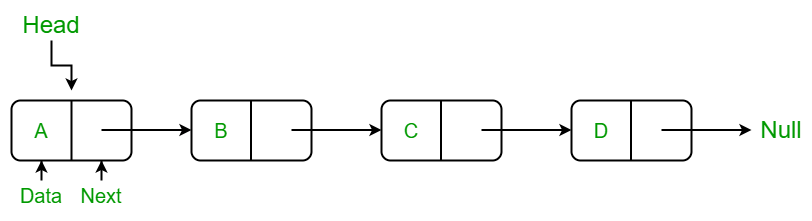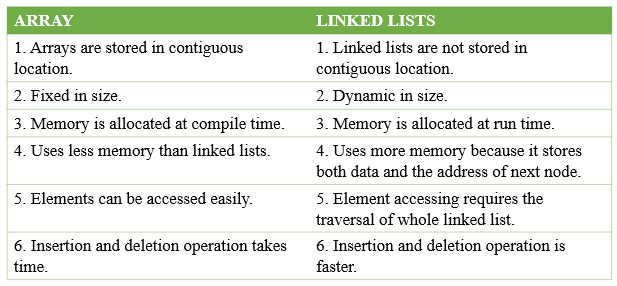GeeksforGeeks App
Open AppBrowser
Continue

## Related Articles

Array: Arrays store elements in contiguous memory locations, resulting in easily calculable addresses for the elements stored and this allows faster access to an element at a specific index.Data storage scheme of an array

Here is the representation of the array in C++:

## C++

 `//c++ program to illustrate arrays in C++``#include // header file for taking input and producing output``using` `namespace` `std;` `int` `main()``{``    ``int` `arr= {1,3,5,3};``//initializing an array of size 4` `    ``cout << arr << ``" "``;``//gives the element at index 3``    ``cout << arr << ``" "``;``//gives the element at index 2` `    ``return` `0;``}``//contributed by Jatin Sharma`

## Python3

 `# Python program to illustrate arrays in Python``arr ``=` `[``1``,``3``,``5``,``3``]``#initializing an array of size 4` `print``(arr[``3``], arr[``2``]) ``#gives the element at index 3 and index 2` `# This code is contributed by akashish__`

## Java

 `public` `class` `Main {``    ``public` `static` `void` `main(String[] args) {``        ``int``[] arr = {``1``, ``3``, ``5``, ``3``}; ``// initializing an array of size 4``        ` `        ``System.out.print(arr[``3``] + ``" "``); ``// gives the element at index 3``        ``System.out.print(arr[``2``] + ``" "``); ``// gives the element at index 2``    ``}``}``// this code is contributed by Tushar Rokade`

## Javascript

 `//Javascript  program to illustrate arrays``//Initializing an array of size 4``const arr = [1,3,5,3];``console.log(arr); ``//gives the element at index 3``console.log(arr); ``//gives the element at index 2``//This code is contributed By Rahul Chauhan`

## C#

 `//C#  program to illustrate arrays``using` `System;` `  ``class` `GFG  {``    ``static` `void` `Main(``string``[] args) {``      ``// Initializing an array of size 4``      ``int``[] arr = {1, 3,5, 3};``      ``Console.WriteLine(arr); ``//gives the element at index 3``      ``Console.WriteLine(arr);``//gives the element at index 2``     ``}``}``//This Code is contributed  By Rahul Chauhan`

Output

`3 5 `

Time Complexity: O(1).
Auxiliary Space: O(1)

Explanation: In the above example we have created an array of size 4 and then accessed the elements using cout of index 3 and 2.

Linked List: Linked lists are less rigid in their storage structure and elements are usually not stored in contiguous locations, hence they need to be stored with additional tags giving a reference to the next element.## C++

 `// Linked list implementation in C++` `#include ``#include ``using` `namespace` `std;` `// Creating a node``class` `Node {``public``:``    ``int` `value;``    ``Node* next;``};` `int` `main()``{``    ``Node* head;``    ``Node* one = NULL;``    ``Node* two = NULL;``    ``Node* three = NULL;` `    ``// allocate 3 nodes in the heap``    ``one = ``new` `Node();``    ``two = ``new` `Node();``    ``three = ``new` `Node();` `    ``// Assign value values``    ``one->value = 1;``    ``two->value = 2;``    ``three->value = 3;` `    ``// Connect nodes``    ``one->next = two;``    ``two->next = three;``    ``three->next = NULL;` `    ``// print the linked list value``    ``head = one;``    ``while` `(head != NULL) {``        ``cout << head->value;``        ``head = head->next;``    ``}``}`

## Java

 `//linked list implementation in java` `public` `class` `Main {``    ``// Creating a Node class``    ``static` `class` `Node {``        ``int` `value;``        ``Node next;``    ``}``    ` `    ``public` `static` `void` `main(String[] args) {``        ``Node head = ``null``;``        ``Node one = ``null``;``        ``Node two = ``null``;``        ``Node three = ``null``;` `        ``// allocate 3 nodes in the heap``        ``one = ``new` `Node();``        ``two = ``new` `Node();``        ``three = ``new` `Node();` `        ``// Assign value values``        ``one.value = ``1``;``        ``two.value = ``2``;``        ``three.value = ``3``;` `        ``// Connect nodes``        ``one.next = two;``        ``two.next = three;``        ``three.next = ``null``;` `        ``// print the linked list value``        ``head = one;``        ``while` `(head != ``null``) {``            ``System.out.print(head.value);``            ``head = head.next;``        ``}``    ``}``}` `//This code is contributed by Tushar Rokade`

## Python3

 `# Linked list implementation in python` `# Creating a node``class` `Node:``    ` `    ``def` `__init__(``self``):``        ``self``.value ``=` `0``        ``self``.``next` `=` `None` `head ``=` `None``one ``=` `None``two ``=` `None``three ``=` `None` `# allocate 3 nodes in the heap``one ``=` `Node()``two ``=` `Node()``three ``=` `Node()` `# Assign value values``one.value ``=` `1``two.value ``=` `2``three.value ``=` `3` `# Connect nodes``one.``next` `=` `two``two.``next` `=` `three``three.``next` `=` `None` `# print the linked list value``head ``=` `one` `while` `(head !``=` `None``):``    ``print``(head.value)``    ``head ``=` `head.``next` `# The code is contributed by Nidhi goel.`

## C#

 `//linked list implementation in C#``using` `System;` `public` `class` `LinkedListNode``{``    ``public` `T Value { ``get``; ``set``; }``    ``public` `LinkedListNode Next { ``get``; ``set``; }` `    ``public` `LinkedListNode(T value)``    ``{``        ``Value = value;``        ``Next = ``null``;``    ``}``}` `public` `class` `LinkedList : System.Collections.Generic.IEnumerable``{``    ``private` `LinkedListNode head;``    ``private` `LinkedListNode tail;` `    ``public` `int` `Count { ``get``; ``private` `set``; }` `    ``public` `void` `AddFirst(T value)``    ``{``        ``var` `node = ``new` `LinkedListNode(value);``        ``if` `(head == ``null``)``        ``{``            ``head = node;``            ``tail = node;``        ``}``        ``else``        ``{``            ``node.Next = head;``            ``head = node;``        ``}``        ``Count++;``    ``}` `    ``public` `void` `AddLast(T value)``    ``{``        ``var` `node = ``new` `LinkedListNode(value);``        ``if` `(tail == ``null``)``        ``{``            ``head = node;``            ``tail = node;``        ``}``        ``else``        ``{``            ``tail.Next = node;``            ``tail = node;``        ``}``        ``Count++;``    ``}` `    ``public` `void` `Remove(T value)``    ``{``        ``if` `(head == ``null``)``        ``{``            ``return``;``        ``}` `        ``if` `(head.Value.Equals(value))``        ``{``            ``head = head.Next;``            ``Count--;``            ``return``;``        ``}` `        ``var` `current = head;``        ``while` `(current.Next != ``null` `&& !current.Next.Value.Equals(value))``        ``{``            ``current = current.Next;``        ``}` `        ``if` `(current.Next != ``null``)``        ``{``            ``current.Next = current.Next.Next;``            ``if` `(current.Next == ``null``)``            ``{``                ``tail = current;``            ``}``            ``Count--;``        ``}``    ``}` `    ``public` `bool` `Contains(T value)``    ``{``        ``var` `current = head;``        ``while` `(current != ``null``)``        ``{``            ``if` `(current.Value.Equals(value))``            ``{``                ``return` `true``;``            ``}``            ``current = current.Next;``        ``}``        ``return` `false``;``    ``}` `    ``public` `void` `Clear()``    ``{``        ``head = ``null``;``        ``tail = ``null``;``        ``Count = 0;``    ``}` `    ``public` `System.Collections.Generic.IEnumerator GetEnumerator()``    ``{``        ``var` `current = head;``        ``while` `(current != ``null``)``        ``{``            ``yield` `return` `current.Value;``            ``current = current.Next;``        ``}``    ``}` `    ``System.Collections.IEnumerator System.Collections.IEnumerable.GetEnumerator()``    ``{``        ``return` `GetEnumerator();``    ``}``}` `public` `class` `Program``{``    ``public` `static` `void` `Main()``    ``{``        ``var` `linkedList = ``new` `LinkedList<``int``>();``        ``linkedList.AddLast(1);``        ``linkedList.AddLast(2);``        ``linkedList.AddLast(3);` `        ``foreach` `(``var` `value ``in` `linkedList)``        ``{``            ``Console.Write(value);``        ``}``    ``}``}``//This Code is Contributed By Rahul Chauhan`

## Javascript

 ``

Output

`123`

Time Complexity: O(1).
Auxiliary Space: O(1)

Explanation: All the struct nodes has a data item and it contains a pointer to the next struct node. It took us only a few steps to create a linked list of three nodes(one, two and three). At first we allocated the nodes and then we assigned values to the node. After assigning the value we connected the nodes from one to next and at the end using while loop printed the entire linked list.

## Major differences between array and linked-list are listed below:

• Size: Since data can only be stored in contiguous blocks of memory in an array, its size cannot be altered at runtime due to the risk of overwriting other data.
However, in a linked list, each node points to the next one such that data can exist at scattered (non-contiguous) addresses; this allows for a dynamic size that can change at runtime.
• Memory allocation: For arrays at compile time and at runtime for linked lists. but, a dynamically allocated array also allocates memory at runtime.
• Memory efficiency: For the same number of elements, linked lists use more memory as a reference to the next node is also stored along with the data. However, size flexibility in linked lists may make them use less memory overall; this is useful when there is uncertainty about size or there are large variations in the size of data elements;
Memory equivalent to the upper limit on the size has to be allocated (even if not all of it is being used) while using arrays, whereas linked lists can increase their sizes step-by-step proportionately to the amount of data.
• Execution time: Any element in an array can be directly accessed with its index. However, in the case of a linked list, all the previous elements must be traversed to reach any element.
Also, better cache locality in arrays (due to contiguous memory allocation) can significantly improve performance. As a result, some operations (such as modifying a certain element) are faster in arrays, while others (such as inserting/deleting an element in the data) are faster in linked lists.
• Insertion: In an array, insertion operation takes more time but in a linked list these operations are fast. For example, if we want to insert an element in the array at the end position in the array and the array is full then we copy the array into another array and then we can add an element whereas if the linked list is full then we find the last node and make it next to the new node
• Dependency: In an array, values are independent of each other but
In the case of linked list nodes are dependent on each other. one node is dependent on its previous node. If the previous node is lost then we can’t find its next subsequent nodes.• The size of the arrays is fixed: So we must know the upper limit on the number of elements in advance. Also, generally, the allocated memory is equal to the upper limit irrespective of usage, and in practical uses, the upper limit is rarely reached.
• Inserting a new element in an array of elements is expensive because a room has to be created for the new elements and to create a room existing elements have to be shifted.

Example:

suppose we maintain a sorted list of IDs in an array id[ ] = [1000, 1010, 1050, 2000, 2040, …..].
And if we want to insert a new ID 1005, then to maintain the sorted order, we have to move all the elements after 1000 (excluding 1000).
Deletion is also expensive with arrays unless some special techniques are used. For example, to delete 1010 in id[], everything after 1010 has to be moved.

1. Dynamic size
2. Ease of insertion/deletion

• Random access is not allowed. We have to access elements sequentially starting from the first node. So we cannot do a binary search with linked lists.
• Extra memory space for a pointer is required for each element of the list.
• Arrays have a better cache locality that can make a pretty big difference in performance.
• It takes a lot of time in traversing and changing the pointers.
• It will be confusing when we work with pointers.

• Arrays store multiple data of similar types with the same name.
• As the array is of fixed size and stored in contiguous memory locations there is no memory shortage or overflow.
• It is helpful to store any type of data with a fixed size.
• Since the elements in the array are stored at contiguous memory locations it is easy to iterate in this data structure and unit time is required to access an element if the index is known.